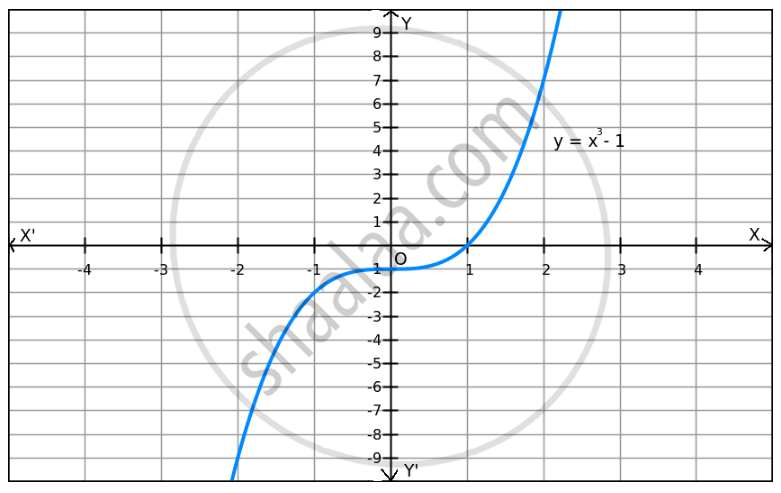Share

F(X) = X3 $-$ 1 on R . - CBSE (Arts) Class 12 - Mathematics

Question

f(x) = x$-$ 1 on R .

SolutionWe can observe that f(x) increases when the values of x increase and f(x) decreases when the values of x decrease.

Also, f(x) can be reduced by giving smaller values of x.

Similarly, f(x) can be enlarged by giving larger values of x.

So, f(x) does not have a minimum or maximum value.

Is there an error in this question or solution?

Video TutorialsVIEW ALL 

Solution F(X) = X3 $-$ 1 on R . Concept: Graph of Maxima and Minima.
S